# CSCI 3328 Object Oriented Programming in C Chapter

• Slides: 12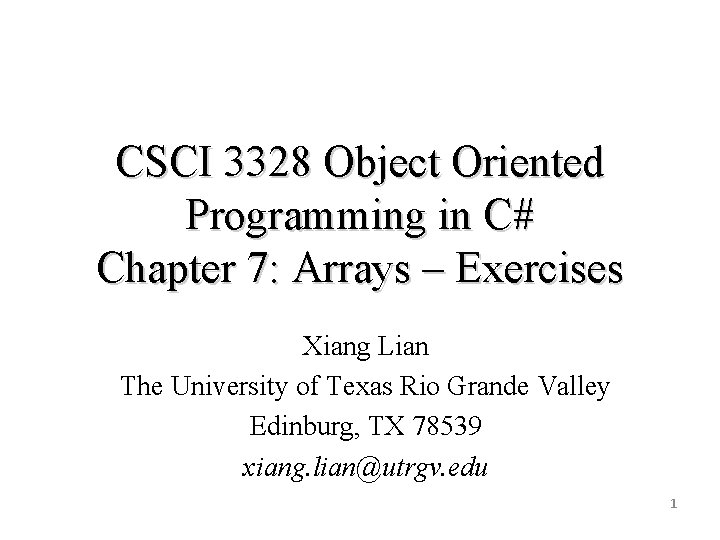CSCI 3328 Object Oriented Programming in C# Chapter 7: Arrays – Exercises Xiang Lian The University of Texas Rio Grande Valley Edinburg, TX 78539 xiang. [email protected] edu 1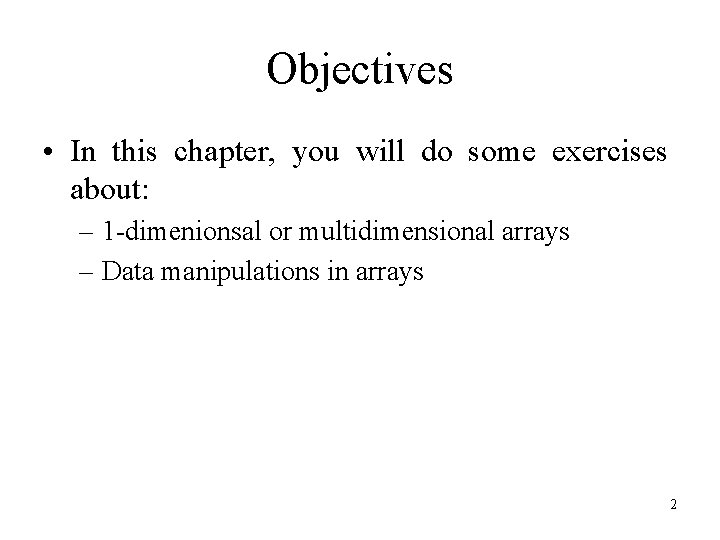Objectives • In this chapter, you will do some exercises about: – 1 -dimenionsal or multidimensional arrays – Data manipulations in arrays 2Multiple Choices • Lists and tables of values with the same data type can be stored in _____. – A. variables B. arrays C. indexes D. keywords • The _____ statement allows you to iterate through the elements in an array without using a counter. – A. for B. each C. for each D. foreach • The number that refers to a particular array element is called the element's _______. – A. number B. data type C. index D. class • An array that uses two indices is referred to as a(n) _____ array. – A. 1 -dimensional B. 2 -dimensional C. matrix D. list • Which of the following statements is true to iterate all elements in the array? – – A. for (int i = 0; i < array. Length; i++) B. for (int i = 1; i < array. Length; i++) C. for (int i = 1; i <= array. Length; i++) D. for (int i = 0; i <= array. Length; i++) 3Multiple Choices (cont'd) • Use the foreach header _____ to iterate through double array numbers. – – A. for each (double d=1; d<numbers. Length; d++) B. foreach (double d = 1 in numbers) C. foreach (double d in numbers) D. for each (double d = numbers[]) • Command-line arguments are stored in _____. – A. string [] args B. string args C. char args D. char [] args • Use the expression _____ to receive the total number of arguments in a command line. – A. args. length B. args. Length() C. args. Get. Length() D. args. Length • The indexed array name of one-dimensional array units's element 2 is _______. – A. units{1} B. units(2) C. units[0, 2] D. units  4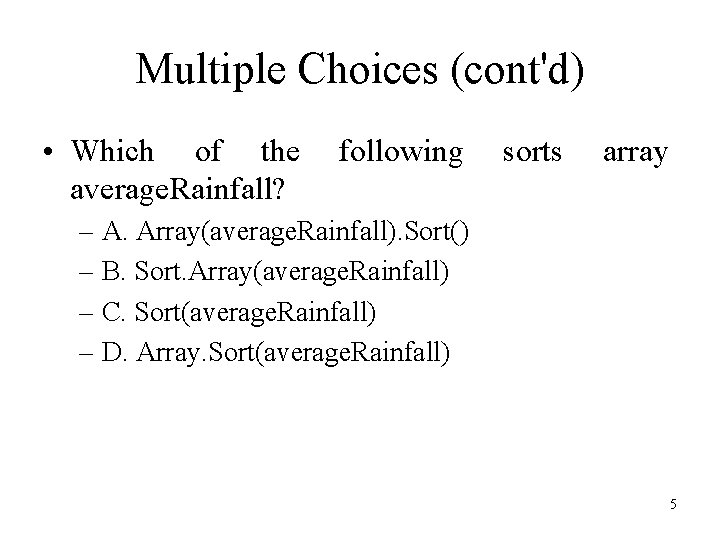Multiple Choices (cont'd) • Which of the average. Rainfall? following sorts array – A. Array(average. Rainfall). Sort() – B. Sort. Array(average. Rainfall) – C. Sort(average. Rainfall) – D. Array. Sort(average. Rainfall) 5True/False Statements • A single array can store values of different types. • An array index should normally be of type float. • An individual array element that is passed to a method and modified in that method will contain the modified value when the called method completes execution. • Command-line arguments are separated by commas. 6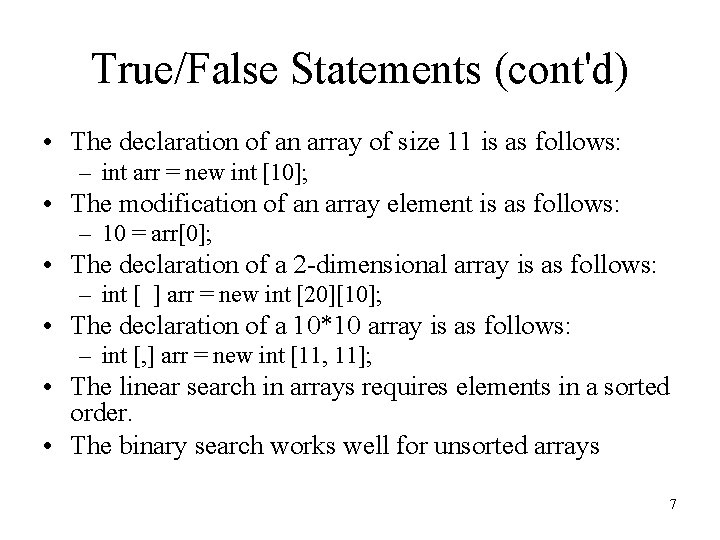True/False Statements (cont'd) • The declaration of an array of size 11 is as follows: – int arr = new int ; • The modification of an array element is as follows: – 10 = arr; • The declaration of a 2 -dimensional array is as follows: – int [ ] arr = new int ; • The declaration of a 10*10 array is as follows: – int [, ] arr = new int [11, 11]; • The linear search in arrays requires elements in a sorted order. • The binary search works well for unsorted arrays 7Debugging Errors const int array_size = 5; array_size = 10; int [] b = new int ; For (int i =0; i<=b. Length; i++) b[i]=1; int [] a = {1, 2, , 4, 5}; b=a; int [, ] c={{1, 2}, {3, 4}}; c = 5; 8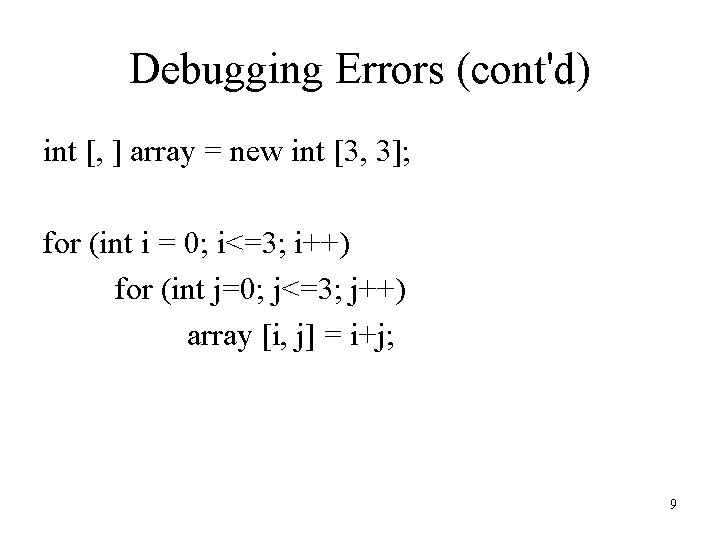Debugging Errors (cont'd) int [, ] array = new int [3, 3]; for (int i = 0; i<=3; i++) for (int j=0; j<=3; j++) array [i, j] = i+j; 9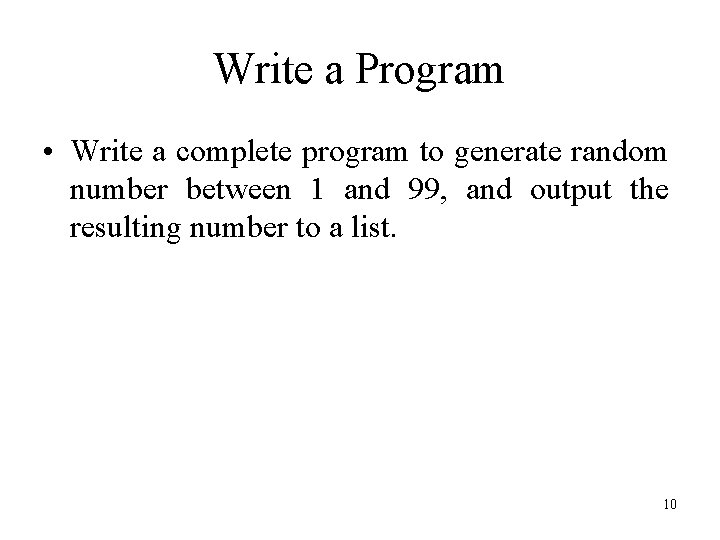Write a Program • Write a complete program to generate random number between 1 and 99, and output the resulting number to a list. 10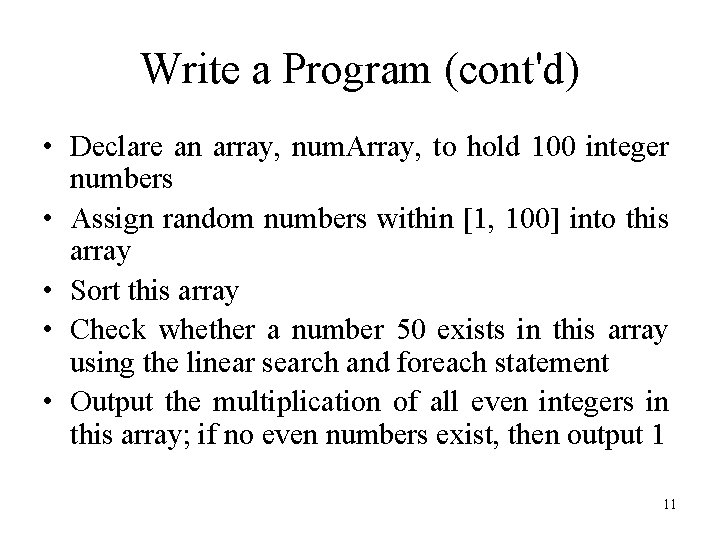Write a Program (cont'd) • Declare an array, num. Array, to hold 100 integer numbers • Assign random numbers within [1, 100] into this array • Sort this array • Check whether a number 50 exists in this array using the linear search and foreach statement • Output the multiplication of all even integers in this array; if no even numbers exist, then output 1 1112